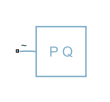# Constant Power Load (Three-Phase)

Three-phase constant power load

• Library:
• Simscape / Electrical / Passive

•## Description

The Constant Power Load (Three-Phase) block implements a constant power load for a three-phase supply.

The block outputs a nominal rated power as long as the voltage from the three-phase supply is equal to or greater than the value specified for the Minimum supply voltage (phase-to-phase RMS) parameter.

When the voltage from the three-phase supply drops below the value of Minimum supply voltage (phase-to-phase RMS), the load behaviour changes and the block models a load with constant impedance.

### Equations

When the voltage from the three-phase supply is greater than the value specified for the Minimum supply voltage (phase-to-phase RMS) parameter, the block acts in constant PQ mode.

The active and reactive power are defined by:

`$\begin{array}{l}P=\frac{3}{2}{V}_{pk}{I}_{pk}cos\left({\phi }_{V}-{\phi }_{I}\right)\\ Q=\frac{3}{2}{V}_{pk}{I}_{pk}\mathrm{sin}\left({\phi }_{V}-{\phi }_{I}\right)\end{array}$`

where:

• Vpk is the voltage peak magnitude.

• ${\phi }_{I}={\phi }_{V}-atan\left(\frac{Q}{P}\right)$ is the phase of the current.

• ${I}_{pk}=\frac{2}{3}\frac{P}{{V}_{pk}cos\left({\phi }_{V}-{\phi }_{I}\right)}$ is the current peak magnitude.

When the voltage from the three-phase supply is less than or equal to the value specified for the Minimum supply voltage (phase-to-phase RMS) parameter, the block acts in constant Z mode.

The active and reactive power are defined by:

`$\begin{array}{l}P=\frac{3}{2}{V}_{pk}^{2}\frac{R}{{R}^{2}+{X}^{2}}\\ Q=\frac{3}{2}{V}_{pk}^{2}\frac{X}{{R}^{2}+{x}^{2}}\end{array}$`

where:

• $R={V}_{line\text{\hspace{0.17em}}RM{S}_{min}}\frac{P}{{P}^{2}+{Q}^{2}}$ is the constant resistance.

• $X={V}_{line\text{\hspace{0.17em}}RM{S}_{min}}\frac{Q}{{P}^{2}+{Q}^{2}}$ is the constant reactance.

• Vline RMSmin is the Minimum supply voltage (phase-to-phase RMS).

If the block is in a network that is compatible with frequency-time simulation mode, you can perform a load-flow analysis on the network. A load-flow analysis provides steady-state values that you can use to initialize a machine.

For more information, see Perform a Load-Flow Analysis Using Simscape Electrical and Frequency and Time Simulation Mode.

## Limitations

If you connect this block to an inductive node or a current source, you must provide a large parasitic.

## Ports

### Conserving

expand all

Expandable three-phase electrical port associated with the three-phase voltage.

## Parameters

expand all

Whether to model composite or expanded three-phase ports.

Composite three-phase ports represent three individual electrical conserving ports with a single block port. You can use composite three-phase ports to build models that correspond to single-line diagrams of three-phase electrical systems.

Expanded three-phase ports represent the individual phases of a three-phase system using three separate electrical conserving ports.

### Main

Active power consumed by the block.

Reactive power consumed by the block.

Minimum voltage, in phase-to-phase RMS, that the supply needs to give the block to act as a constant power load.

### Faults

Select `On` to enable faults modeling and expose the associated parameters in the Faults section, which enables you to select the reporting method and specify the time at which the fault triggers.

Choose whether to issue an assertion when a fault occurs:

• `None` — The block does not issue an assertion.

• `Warn` — The block issues a warning.

• `Error` — The simulation stops with an error.

#### Dependencies

To enable this parameter, set Enable open circuit fault to `On`.

Simulation time when the block enters the faulted state.

#### Dependencies

To enable this parameter, set Enable open circuit fault to `On`.

Conductance during the open-circuit state.

#### Dependencies

To enable this parameter, set Enable open circuit fault to `On`.

## Version History

Introduced in R2020b